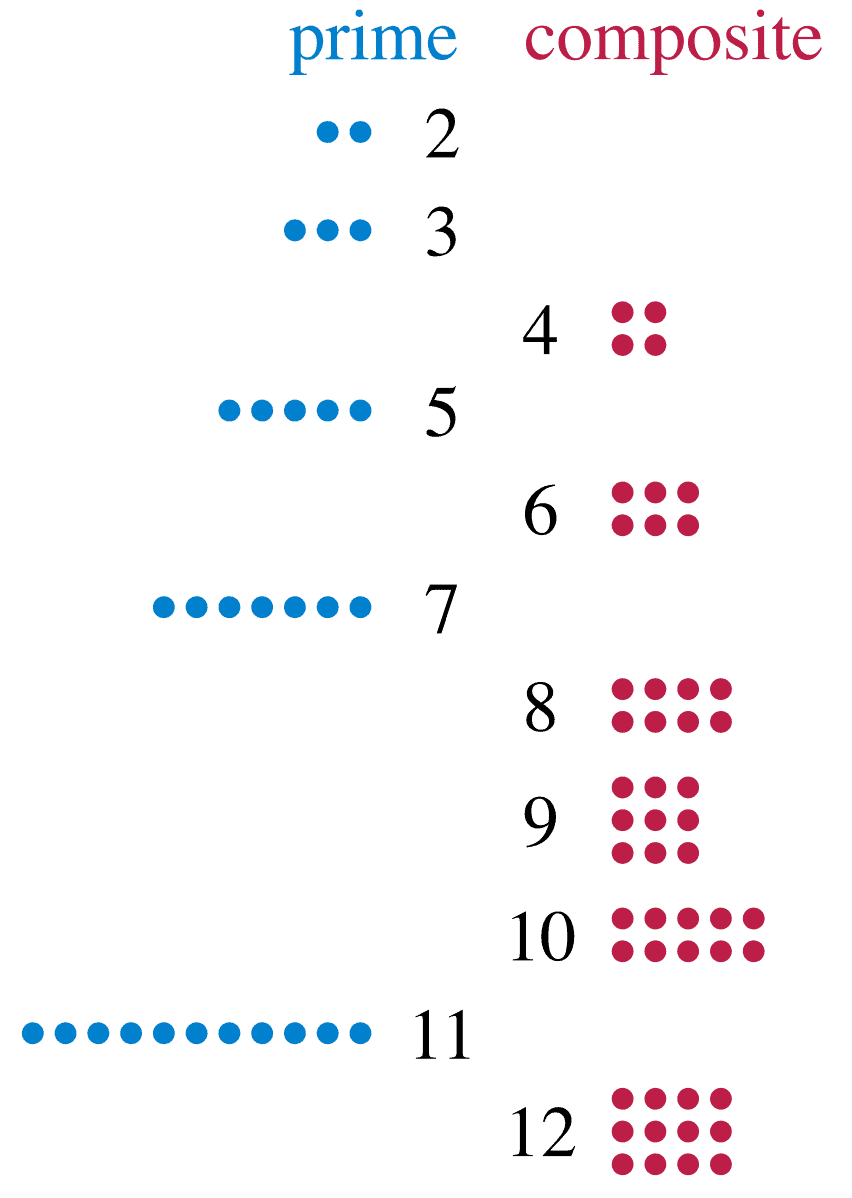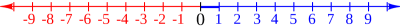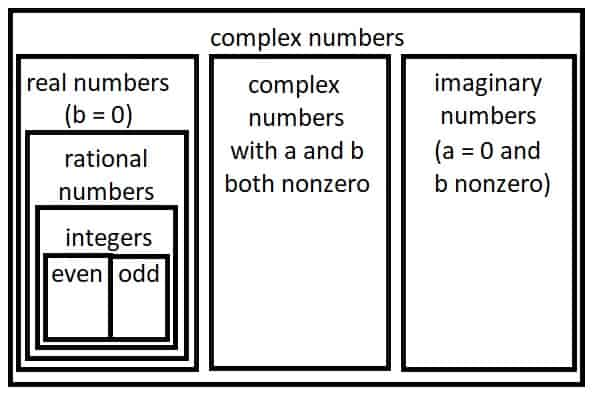# The Number Zero (12 Common Questions Answered)

Zero is an important and necessary concept in mathematics.  However, there are still many questions about this unique number.

So, what do you need to know about the number zero?  Zero is an even number, but it is neither prime nor composite. Zero is neither positive nor negative, but it bridges the gap between them. Zero factorial is 1 (0! = 1). Zero is real, rational, and an integer, but it is not a natural number. Division by zero is undefined, as is zero divided by zero.

Of course, the number zero did not always exist.  After all, why talk about something if you have none of it?

Let’s get started.

## The Number Zero

The number zero had to be invented, since it is not a natural concept.  After all, if you have zero of something, why bother talking about it at all?

Zero is important in the decimal number system (base 10), and also in computers (where the binary digit zero means “no charge”, “no”, or “false”.

### Is Zero A Prime Number?

Zero is not a prime number.  We can see this from the definition of a prime number:

• A prime number is a natural number greater than 1 that is not a product of two smaller natural numbers.

Since 0 is not greater than 1, it cannot be a prime number.  We can also use another fact about prime numbers to show that zero is not prime:Zero is not a prime number, since the definition requires a prime number to be a positive integer.
• A prime number has exactly two positive factors: 1 and itself.

For example, the number 7 is prime because it has 2 nonnegative factors: 1 and 7.  This means we can write 7 as the product of two nonnegative factors: 7 = 1*7

However, 9 is not prime because it has 3 nonnegative factors: 1, 3, and 9.  We can write it as 9 = 1*9 or 9 = 3*3.

Zero is not prime because we can write is as a product of more than two nonnegative factors.  For example:

• 0 = 0*1*2  (3 nonnegative factors)
• 0 = 0*2*4*6 (4 nonnegative factors)
• 0 = 0*5*10*15*20 (5 nonnegative factors)

Note: by similar reasoning, 1 is not a prime number either.

### Is Zero A Composite Number?

Zero is not a composite number.  We can see this from the definition of a composite number:

• A composite number is a positive integer that can be written as the product of two smaller positive integers.

Since 0 is not positive, it cannot be a composite number.

Note: by this definition, 1 is also not composite, since it cannot be written as a product of two smaller positive integers.

### Is Zero An Even Number?

Zero is an even number, since the remainder after dividing by 2 is zero.  In other words, zero is a multiple of 2 (since 0 = 2*0).

We can also see that zero is even by listing out the multiples of 2 (even numbers):

• …, -6, -4, -2, 0, 2, 4, …

As multiples of 2, the even numbers are:

• …, 2*(-3), 2*(-2), 2*(-1), 2*(0), 2*(1), 2*(2), 2*(3), …

Another way to see that zero is even is to think about a sum of even numbers.  The sum of any two even numbers is also even.

Since 2 and -2 are both even, then 2 + (-2) = 0 is also even.

### Why Is Zero Factorial 1?

Zero factorial is 1 (0! = 1) by convention, as a sort of “base case”.  However, we can also prove that 0! “should” equal 1 by examining the following table.

To get from one row in the table to the next, we divide by n.  For example, to go from 5! To 4!, we divide by 5 (120 / 5 = 24).

To go from 4! to 3!, we divide by 4 (24 / 6 = 4). To go from 3! to 2!, we divide by 3 (6 / 3 = 2). To go from 2! to 1!, we divide by 2 (2 / 2 = 1).

So, to go from 1! To 0!, we divide by 1.  Since 1 / 1 = 1, we can see that 0! = 1.

### Is Zero A Positive Number?

Zero is not a positive number.  By definition, a positive number is greater than 0.

Zero cannot be positive, since a number cannot be greater than itself (that is, 0 > 0 is impossible).

As a result, we can say that zero is a nonpositive number (less than or equal to zero).

### Is Zero A Negative Number?

Zero is not a negative number.  By definition, a negative number is less than 0.

Zero cannot be negative, since a number cannot be less than itself (that is, 0 < 0 is impossible).

As a result, we can say that zero is a nonnegative number (greater than or equal to zero).

### What Is The Absolute Value Of Zero?

The absolute value of zero is zero.  This is because the absolute value of x (or |x|) is defined as:

• The nonnegative value of x without regard to sign

(zero has no sign, so it doesn’t change under absolute value).

In other words, for a nonnegative number x, we have |x|= x.  Since x = 0 is nonnegative, the absolute value of zero is zero.

We can also think of the absolute value of x as “the distance on the number line from 0 to x”.The absolute value of x is the distance from 0 to x on the number line.

For example, the absolute value of 2 is 2, since it is a distance of 2 units away from zero on the number line.

Similarly, the absolute value of -2 is 2, since it is a distance of 2 units away from zero on the number line.

Then the absolute value of zero is zero, since zero is a distance of 0 units away from itself on the number line.

### Is Zero A Natural Number?

Zero is not a natural number.  The set of natural numbers is the set of positive integers (whole numbers greater than zero, without fractions or decimals):

• 1, 2, 3, 4, 5, 6, …

However, zero is a whole number, which is the set of natural numbers along with zero:

• 0, 1, 2, 3, 4, 5, 6, …

### Is Zero An Integer?

Zero is an integer, since zero is a whole number, and every whole number is an integer.  We can write zero without any fraction or decimal part, so it is an integer.

We can think of the set of integers as the set of natural numbers (1, 2, 3, 4, 5, 6, …) together with their additive inverses (-1, -2, -3, -4, -5, -6, …) and the number zero (0).

### Is Zero A Rational Number?

Zero is a rational number, since zero is an integer, and any integer is a rational number.  We can write zero as a rational number with any denominator we wish.

For example, 0 = 0 / 1, or 0 = 0 / 2, and so on.

### Is Zero A Real Number?

Zero is a real number, since zero is a rational number, and any rational number is a real number.  In fact, the set of real numbers is the union of the set of rational numbers with the set of irrational numbers.

Zero is important because it is additive identity element for the integers, rational numbers, and real numbers.  That is, x + 0 = x = 0 + x for any real number x.Every even or odd number is an integer, every integer is a rational number, and every rational number is a real number.

### Can You Divide By Zero?

You cannot divide by zero, since a zero denominator is undefined.  On the graph of a function, division by zero means that we have a vertical asymptote.

For example, the rational function y = 1 / x has a vertical asymptote at x = 0.

[graph of y = 1 / x]

A rational function can have more than one vertical asymptote.  For example, the rational function y = 1 / (x – 2)(x – 4) has vertical asymptotes at x = 2 and x = 4.

#### What Is Zero Divided By Zero?

Zero divided by zero is undefined, since it involves division by zero.  If we get a result of zero divided by zero when taking a limit, then we call this an indeterminate form.

We would need to do more work to determine what the limit is.

For example, limx->1 (x – 1) / (x2 – 1) appears to give us a limit of 0 / 0 (if we plug in x = 1 on the top and bottom).  However, if we factor the denominator as a difference of squares, we get:

• (x – 1) / (x2 – 1)
• =(x – 1) / (x + 1)(x – 1)  [factor (x2 – 1) as a difference of squares]
• =1 / (x + 1)  [cancel the (x – 1) in the numerator and denominator]

This gives us a limit of limx->1 (x – 1) / (x2 – 1) = limx->1 1 / (x + 1) = ½.